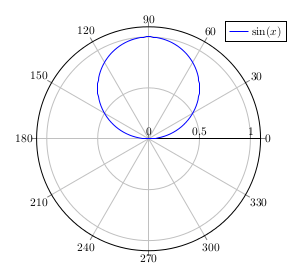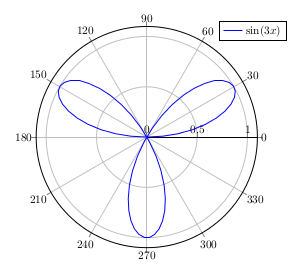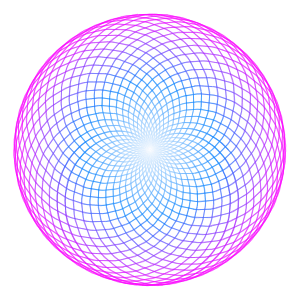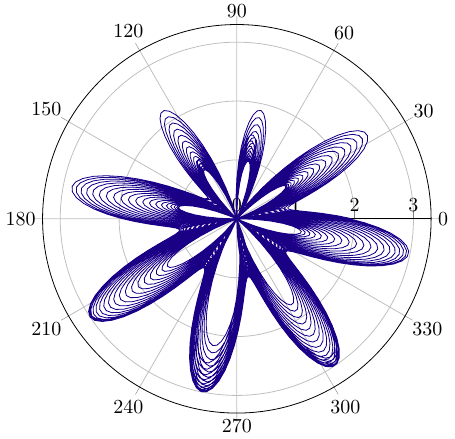# Periodically, more or less

That’s from my German blog TikZ.de.Recently I played with the sine function, that “wave” that everybody knows in cartesian coordinates. Let’s take a look at a 3d polar complex sine made plot.

In polar coordinates the sine function is a simple circle:

\documentclass[border=10pt]{standalone}
\usepackage{pgfplots}
\usepgfplotslibrary{polar}
\begin{document}
\begin{tikzpicture}
\begin{polaraxis}[
domain  = 0:180,
samples = 100,
]
\legend{$\sin(x)$}
\end{polaraxis}
\end{tikzpicture}
\end{document}When we shorten the period length, we get:We can take a rational factor:

\documentclass{standalone}
\usepackage{pgfplots}
\usepgfplotslibrary{polar,colormaps}
\begin{document}
\begin{tikzpicture}
\begin{polaraxis}[
domain  = -14400:14400,
samples = 3000,
colormap/cool,
hide axis
]
\end{polaraxis}
\end{tikzpicture}
\end{document}By adding another sine with other factors, we get more movement:

\documentclass{standalone}
\usepackage{pgfplots}
\usepgfplotslibrary{polar}
\begin{document}
\begin{tikzpicture}
\begin{polaraxis}[
domain = -3600:3600,
samples = 4000
]
\addplot[blue!50!black] {1 - sin(50*x/49) - sin(8*x)};
\end{polaraxis}
\end{tikzpicture}
\end{document}Let’s have a 3d view with growing angle.

We make a parametrical 3d-Plot in x and y: x runs the circle from -180 to 180 degree, we make a sampling for y for the number of rotations. We add y time 360 degrees to the function argument. y is our third dimension, while x as angle and the function value are the the original two dimensions.

\documentclass[border=10pt]{standalone}
\usepackage{pgfplots}
\begin{document}
\begin{tikzpicture}
\begin{axis}[
domain    = -180:180,
y domain  = -19:19,
samples y = 39,
samples   = 100,
z buffer  = sort,
colormap/cool,
grid
]
\end{document}